# NCERT solutions for Class 12 Physics Textbook chapter 3 - Current Electricity [Latest edition]

#### Chapters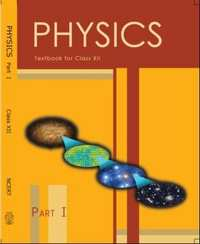## Chapter 3: Current Electricity

Exercise
Exercise [Pages 127 - 130]

### NCERT solutions for Class 12 Physics Textbook Chapter 3 Current Electricity Exercise [Pages 127 - 130]

Exercise | Q 1 | Page 127

The storage battery of a car has an emf of 12 V. If the internal resistance of the battery is 0.4 Ω, what is the maximum current that can be drawn from the battery?

Exercise | Q 2 | Page 127

A battery of emf 10 V and internal resistance 3 Ω is connected to a resistor. If the current in the circuit is 0.5 A, what is the resistance of the resistor? What is the terminal voltage of the battery when the circuit is closed?

Exercise | Q 3.1 | Page 127

Three resistors 1 Ω, 2 Ω, and 3 Ω are combined in series. What is the total resistance of the combination?

Exercise | Q 3.2 | Page 127

If the combination is connected to a battery of emf 12 V and negligible internal resistance, obtain the potential drop across each resistor.

Exercise | Q 4.1 | Page 127

Three resistors 2 Ω, 4 Ω and 5 Ω are combined in parallel. What is the total resistance of the combination?

Exercise | Q 4.2 | Page 127

If the combination is connected to a battery of emf 20 V and negligible internal resistance, determine the current through each resistor, and the total current drawn from the battery.

Exercise | Q 5 | Page 127

At room temperature (27.0°C) the resistance of a heating element is 100 Ω. What is the temperature of the element if the resistance is found to be 117 Ω, given that the temperature coefficient of the material of the resistor is 1.70 × 10−4 °C−1.

Exercise | Q 6 | Page 127

A negligibly small current is passed through a wire of length 15 m and uniform cross-section 6.0 × 10−7 m2, and its resistance is measured to be 5.0 Ω. What is the resistivity of the material at the temperature of the experiment?

Exercise | Q 7 | Page 127

A silver wire has a resistance of 2.1 Ω at 27.5°C, and a resistance of 2.7 Ω at 100°C. Determine the temperature coefficient of resistivity of silver.

Exercise | Q 8 | Page 127

A heating element using nichrome connected to a 230 V supply draws an initial current of 3.2 A which settles after a few seconds to a steady value of 2.8 A. What is the steady temperature of the heating element if the room temperature is 27.0°C? Temperature coefficient of resistance of nichrome averaged over the temperature range involved is 1.70 × 10−4 °C−1.

Exercise | Q 9 | Page 128

Determine the current in each branch of the network shown in fig.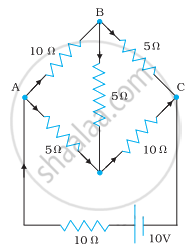Exercise | Q 10 | Page 128

(a) In a metre bridge [shown in the figure], the balance point is found to be at 39.5 cm from the end A, when the resistor Y is 12.5 Ω. Determine the resistance of X. Why are the connections between resistors in a Wheatstone or meter bridge made of thick copper strips?

(b) Determine the balance point of the bridge above if X and Y are interchanged.

(c) What happens if the galvanometer and cell are interchanged at the balance point of the bridge? Would the galvanometer show any current?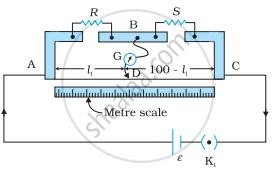Exercise | Q 11 | Page 128

A storage battery of emf 8.0 V and internal resistance 0.5 Ω is being charged by a 120 V dc supply using a series resistor of 15.5 Ω. What is the terminal voltage of the battery during charging? What is the purpose of having a series resistor in the charging circuit?

Exercise | Q 12 | Page 128

In a potentiometer arrangement, a cell of emf 1.25 V gives a balance point at 35.0 cm length of the wire. If the cell is replaced by another cell and the balance point shifts to 63.0 cm, what is the emf of the second cell?

Exercise | Q 13 | Page 128

The number density of free electrons in a copper conductor estimated in Example 3.1 is 8.5 × 1028 m−3. How long does an electron take to drift from one end of a wire 3.0 m long to its other end? The area of cross-section of the wire is 2.0 × 10−6 m2 and it is carrying a current of 3.0 A.

Exercise | Q 14 | Page 128

The earth’s surface has a negative surface charge density of 10−9 C m−2. The potential difference of 400 kV between the top of the atmosphere and the surface results (due to the low conductivity of the lower atmosphere) in a current of only 1800 A over the entire globe. If there were no mechanism of sustaining atmospheric electric field, how much time (roughly) would be required to neutralise the earth’s surface? (This never happens in practice because there is a mechanism to replenish electric charges, namely the continual thunderstorms and lightning in different parts of the globe). (Radius of earth = 6.37 × 106 m.)

Exercise | Q 15.1 | Page 129

A secondary cell after long use has an emf of 1.9 V and a large internal resistance of 380 Ω. What maximum current can be drawn from the cell? Could the cell drive the starting motor of a car?

Exercise | Q 15.1 | Page 129

Six lead-acid types of secondary cells each of emf 2.0 V and internal resistance 0.015 Ω are joined in series to provide a supply to a resistance of 8.5 Ω. What are the current drawn from the supply and its terminal voltage?

Exercise | Q 16 | Page 129

Two wires of equal length, one of aluminium and the other of copper have the same resistance. Which of the two wires is lighter? Hence explain why aluminium wires are preferred for overhead power cables.
Al = 2.63 × 10−8 Ω m, ρCu = 1.72 × 10−8 Ω m, Relative density of Al = 2.7, of Cu = 8.9.)

Exercise | Q 17 | Page 129

What conclusion can you draw from the following observations on a resistor made of alloy manganin?

 CurrentA VoltageV CurrentA VoltageV 0.2 3.94 3.0 59.2 0.4 7.87 4.0 78.8 0.6 11.8 5.0 98.6 0.8 15.7 6.0 118.5 1.0 19.7 7.0 138.2 2.0 39.4 8.0 158.0
Exercise | Q 18.1 | Page 129

A steady current flows in a metallic conductor of non-uniform cross-section. Which of these quantities is constant along the conductor: current, current density, electric field, drift speed

Exercise | Q 18.2 | Page 129

Is Ohm’s law universally applicable for all conducting elements? If not, give examples of elements which do not obey Ohm’s law.

Exercise | Q 18.3 | Page 129

A low voltage supply from which one needs high currents must have very low internal resistance. Why?

Exercise | Q 18.4 | Page 129

A high tension (HT) supply of, say, 6 kV must have a very large internal resistance. Why?

Exercise | Q 19.1 | Page 129

Choose the correct alternative:

Alloys of metals usually have (greater/less) resistivity than that of their constituent metals.

Exercise | Q 19.2 | Page 129

Choose the correct alternative:

Alloys usually have much (lower/higher) temperature coefficients of resistance than pure metals.

Exercise | Q 19.3 | Page 129

Choose the correct alternative:

The resistivity of the alloy manganin is nearly independent of/increases rapidly with increase of temperature.

Exercise | Q 19.4 | Page 129

Choose the correct alternative:

The resistivity of a typical insulator (e.g., amber) is greater than that of a metal by a factor of the order of (1022/1023).

Exercise | Q 20 | Page 129

Given the resistances of 1 Ω, 2 Ω, 3 Ω, how will be combine them to get an equivalent resistance of (11/3) Ω?

Exercise | Q 21 | Page 130

Determine the current drawn from a 12 V supply with internal resistance 0.5 Ω by the infinite network shown in the figure. Each resistor has 1 Ω resistance.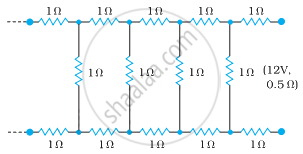Exercise | Q 22 | Page 130

Figure shows a potentiometer with a cell of 2.0 V and internal resistance 0.40 Ω maintaining a potential drop across the resistor wire AB. A standard cell which maintains a constant emf of 1.02 V (for very moderate currents up to a few mA) gives a balance point at 67.3 cm length of the wire. To ensure very low currents drawn from the standard cell, very high resistance of 600 kΩ is put in series with it, which is shorted close to the balance point. The standard cell is then replaced by a cell of unknown emf ε and the balance point found similarly, turns out to be at 82.3 cm length of the wire.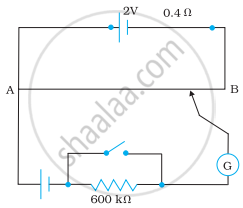(a) What is the value ε?

(b) What purpose does the high resistance of 600 kΩ have?

(c) Is the balance point affected by this high resistance?

(d) Is the balance point affected by the internal resistance of the driver cell?

(e) Would the method work in the above situation if the driver cell of the potentiometer had an emf of 1.0 V instead of 2.0 V?

(f) Would the circuit work well for determining an extremely small emf, say of the order of a few mV (such as the typical emf of a thermo-couple)? If not, how will you modify the circuit?

Exercise | Q 23 | Page 130

Figure shows a 2.0 V potentiometer used for the determination of internal resistance of a 1.5 V cell. The balance point of the cell in open circuit is 76.3 cm. When a resistor of 9.5 Ω is used in the external circuit of the cell, the balance point shifts to 64.8 cm length of the potentiometer wire. Determine the internal resistance of the cell.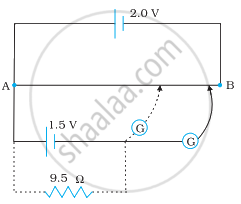## Chapter 3: Current Electricity

Exercise## NCERT solutions for Class 12 Physics Textbook chapter 3 - Current Electricity

NCERT solutions for Class 12 Physics Textbook chapter 3 (Current Electricity) include all questions with solution and detail explanation. This will clear students doubts about any question and improve application skills while preparing for board exams. The detailed, step-by-step solutions will help you understand the concepts better and clear your confusions, if any. Shaalaa.com has the CBSE Class 12 Physics Textbook solutions in a manner that help students grasp basic concepts better and faster.

Further, we at Shaalaa.com provide such solutions so that students can prepare for written exams. NCERT textbook solutions can be a core help for self-study and acts as a perfect self-help guidance for students.

Concepts covered in Class 12 Physics Textbook chapter 3 Current Electricity are Limitations of Ohm’s Law, Electric Currents in Conductors, Conductivity and Conductance;, Current Density, Delta Star Transformation, Potential Difference and Emf of a Cell, Measurement of Internal Resistance of a Cell, Potentiometer, Metre Bridge, Wheatstone Bridge, Kirchhoff’s Rules, Combination of Cells in Series and in Parallel, Cells, Emf, Internal Resistance, Temperature Dependence of Resistance, Combination of Resistors - Series and Parallel, Resistivity of Various Materials, Electrical Resistivity and Conductivity, V-I Characteristics (Linear and Non-linear), Drift of Electrons and the Origin of Resistivity, Flow of Electric Charges in a Metallic Conductor, Electric Current, Ohm's Law, Electrical Power.

Using NCERT Class 12 solutions Current Electricity exercise by students are an easy way to prepare for the exams, as they involve solutions arranged chapter-wise also page wise. The questions involved in NCERT Solutions are important questions that can be asked in the final exam. Maximum students of CBSE Class 12 prefer NCERT Textbook Solutions to score more in exam.

Get the free view of chapter 3 Current Electricity Class 12 extra questions for Class 12 Physics Textbook and can use Shaalaa.com to keep it handy for your exam preparation